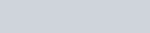nuclear equation Multiple choice questions, chemistry homework help
1. At this point, please review your notes to prepare to take a summative practice.This summative practice will check your understanding of the concepts discussed in this portion of your unit. If you pass this practice, you will be able to move forward to the next portion of the unit. If you do not pass, you will be asked to complete a reteach or remediation activity depending on your grade before being able to retake this test and move forward.

Complete the following nuclear equation:1. The mass of the nucleus is

greater than the mass of the protons and neutrons that make up the nucleus.

equal to the mass of the protons and neutrons that make up the nucleus. less than the mass of the protons and neutrons that make up the nucleus.

converted to energy.

1. Which type of radiation has the most penetrating ability?

an alpha particle a beta particle a gamma ray a neutron

1. Which two particles have the same mass but opposite charge?

A beta particle and a positron

A neutron and a proton

A proton and an electron

An alpha particle and a proton

1. Which of the following nuclear equations is correctly balanced?1. Gamma rays

have the same energy as beta particles do. are visible light. have no charge and no mass.

are not a form of electromagnetic radiation

1. Which of the following nuclides is radioactive?1. The half-life of thorium-234 is 24 days. If you have a 42.0 g sample of thorium-24, how much will remain after 72 days?

42.0 g

21.0 g

10.5 g

5.25 g

1. It takes 5.2 min for a 4.0 g sample of francium-210 to decay until only 1.0 g is left. What is the half-life of francium-210?

1.3 min

2.6 min

5.2 min

7.8 min